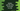# Python index method to get the index of an item in a list### Python index method to get the index of an item in a list :

The ‘index’ method is used to find the index of the first occurrence of an item in a list. The syntax of ‘index’ method is as below :

``````s.index(x[, i[, j]])
``````

It will find the index of the first occurrence of ‘x’ in list ‘s’. ‘i’ and ‘j’ are optional index numbers. If given, it will find the first occurrence after index ‘i’ and before index ‘j’. Let’s take a look :

``````my_list = ['a','b','d','e','f','z','b','k']

print(my_list.index('b'))
``````

In this program, for the list ‘my_list’, we are printing the first occurrence of ‘b’. Since ‘b’ is in the second position, it’s index is ‘1’. (index starts from ‘0’). So, the program will print ‘1’. What will be the output of the below program?

``````my_list = ['a','b','d','e','f','z','b','k']

print(my_list.index('b',2))
``````

Here, we are passing one more parameter ‘2’ in the ‘index()’ method. That means it will check for the index of ‘b’ starting from index ‘2’. Since ‘b’ is also in the seventh position, it will print ‘6’.

``````my_list = ['a','b','d','e','f','z','b','k']

print(my_list.index('b',2,5))
``````

The output of the above program is :

``````ValueError: 'b' is not in list
``````

Here we are passing two more arguments with ‘b’ to ‘index’ method. It will check for the first occurrence of ‘b’ after the first argument i.e. 2 and before the second argument i.e. 5. Since there is no ‘b’ within ‘2’ and ‘5’, it will throw ‘ValueError’ .